Statistical Assumptions for Using PROC GLM

The basic statistical assumption underlying the least squares approach to general linear modeling is that the observed values of each dependent variable can be written as the sum of two parts: a fixed component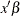, which is a linear function of the independent coefficients, and a random noise, or error, component: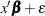The independent coefficients x are constructed from the model effects as described in the section Parameterization of PROC GLM Models. Further, the errors for different observations are assumed to be uncorrelated with identical variances. Thus, this model can be written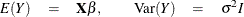where Y is the vector of dependent variable values,is the matrix of independent coefficients, I is the identity matrix, andis the common variance for the errors. For multiple dependent variables, the model is similar except that the errors for different dependent variables within the same observation are not assumed to be uncorrelated. This yields a multivariate linear model of the form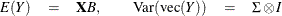where Y and B are now matrices, with one column for each dependent variable,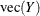strings Y out by rows, and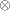indicates the Kronecker matrix product.

Under the assumptions thus far discussed, the least squares approach provides estimates of the linear parameters that are unbiased and have minimum variance among linear estimators. Under the further assumption that the errors have a normal (or Gaussian) distribution, the least squares estimates are the maximum likelihood estimates and their distribution is known. All of the significance levels (p values) and confidence limits calculated by the GLM procedure require this assumption of normality in order to be exactly valid, although they are good approximations in many other cases.